## Alexander Polynomial

A Polynomial invariant of a Knot discovered in 1923 by J. W. Alexander (Alexander 1928). In technical language, the Alexander polynomial arises from the Homology of the infinitely cyclic cover of a Knot's complement. Any generator of a Principal Alexander Ideal is called an Alexander polynomial (Rolfsen 1976). Because the Alexander Invariant of a Tame Knot inhas a Square presentation Matrix, its Alexander Ideal is Principal and it has an Alexander polynomial denoted.

Letbe the Matrix Product of Braid Words of a Knot, then(1)

where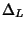is the Alexander polynomial and det is the Determinant. The Alexander polynomial of a Tame Knot insatisfies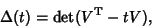(2)

whereis a Seifert Matrix, det is the Determinant, anddenotes the Matrix Transpose. The Alexander polynomial also satisfies(3)

The Alexander polynomial of a splittable link is always 0. Surprisingly, there are known examples of nontrivial Knots with Alexander polynomial 1. An example is the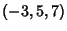Pretzel Knot.

The Alexander polynomial remained the only known Knot Polynomial until the Jones Polynomial was discovered in 1984. Unlike the Alexander polynomial, the more powerful Jones Polynomial does, in most cases, distinguish Handedness. A normalized form of the Alexander polynomial symmetric in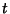andand satisfying(4)

was formulated by J. H. Conway and is sometimes denoted. The Notationis an abbreviation for the Conway-normalized Alexander polynomial of a Knot(5)

For a description of the Notation for Links, see Rolfsen (1976, p. 389). Examples of the Conway-Alexander polynomials for common Knots include(6)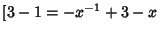(7)(8)

for the Trefoil Knot, Figure-of-Eight Knot, and Solomon's Seal Knot, respectively. Multiplying through to clear the Negative Powers gives the usual Alexander polynomial, where the final Sign is determined by convention.Let an Alexander polynomial be denoted, then there exists a Skein Relationship (discovered by J. H. Conway)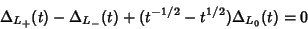(9)

corresponding to the above Link Diagrams (Adams 1994). A slightly different Skein Relationship convention used by Doll and Hoste (1991) is(10)

These relations allow Alexander polynomials to be constructed for arbitrary knots by building them up as a sequence of over- and undercrossings.

For a Knot,(11)

where Arf is the Arf Invariant (Jones 1985). Ifis a Knot and(12)

thencannot be represented as a closed 3-Braid. Also, if(13)

thencannot be represented as a closed 4-braid (Jones 1985).

The HOMFLY Polynomial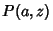generalizes the Alexander polynomial (as well at the Jones Polynomial) with(14)

(Doll and Hoste 1991).

Rolfsen (1976) gives a tabulation of Alexander polynomials for Knots up to 10 Crossings and Links up to 9 Crossings.

References

Adams, C. C. The Knot Book: An Elementary Introduction to the Mathematical Theory of Knots. New York: W. H. Freeman, pp. 165-169, 1994.

Alexander, J. W. Topological Invariants of Knots and Links.'' Trans. Amer. Math. Soc. 30, 275-306, 1928.

Alexander, J. W. A Lemma on a System of Knotted Curves." Proc. Nat. Acad. Sci. USA 9, 93-95, 1923.

Doll, H. and Hoste, J. A Tabulation of Oriented Links.'' Math. Comput. 57, 747-761, 1991.

Jones, V. A Polynomial Invariant for Knots via von Neumann Algebras.'' Bull. Amer. Math. Soc. 12, 103-111, 1985.

Rolfsen, D. Table of Knots and Links.'' Appendix C in Knots and Links. Wilmington, DE: Publish or Perish Press, pp. 280-287, 1976.

Stoimenow, A. Alexander Polynomials.'' http://www.informatik.hu-berlin.de/~stoimeno/ptab/a10.html.

Stoimenow, A. Conway Polynomials.'' http://www.informatik.hu-berlin.de/~stoimeno/ptab/c10.html.